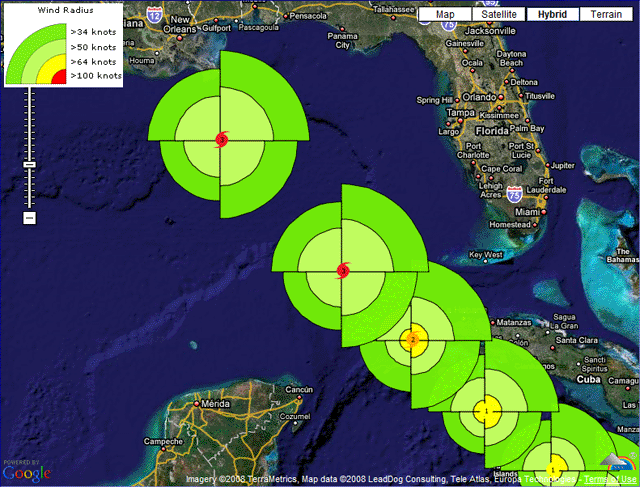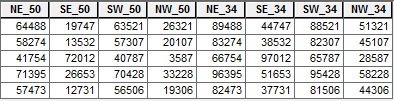# Is it possible to make a buffer that has four quadrants with different radii?

2737
19
12-16-2016 04:24 PMNew Contributor II

Hello,

I couldn't find an answer to this in previous discussions so hopefully I'm asking a new question.

I am using ArcMap 10.2.2 and I have "extended best track" data for tropical cyclones which has latitude and longitude locations of storms at 6 hour intervals as well as estimated wind radii for the NE, SE, SW, and NW quadrants at those locations. For instance, at lat x long y, this data might have estimated radius for a 64 kt wind of 100 nautical miles in the NE quadrant, 75 nm to the SE, etc.

So basically, I want to buffer these point locations with quadrant specific radii based on the attribute for that quadrant. Is this possible to do? I'm not well versed in coding but I would be willing to try to figure it out if it is possible and can be automated either with python or model builder to do for multiple storms. Any suggestions would be much appreciated.

Thanks,

Pat

Tags (1)
19 RepliesOccasional Contributor III

Could you draw a picture for what you want to do?New Contributor II

Sorry, I just realized I didn't actually reply to you, I replied to the post. Please refer to the picture in the post below. Thanks for your help.New Contributor II

Sure thing. I found this picture online that shows almost exactly what I want to do:Ignoring the different colors which would be like different layers based on wind speed, I'm wondering if it's possible to create buffers around a point that have different radii per quadrant. The radius length for each quadrant (NE, SE, SW, and NW) would be indicated in an attribute table.Occasional Contributor III

I know that the thread is relate to symbology.

But I think it is difficult to create that symbol using symbology in Arc Map.

So I will try to make this as a features (and you can convert it to graphic later)

I can say that your symbols can be done, Using model builder.

And,How many points do you have?New Contributor II

Thanks for the response, Abdullah. I have a lot of points - almost 5,000 in

total. I have a school site license so I do not think it's an advanced

license. Hopefully something can still be done with that information.

ThanksbyMVP Honored Contributor

You'll probably have to do some tweaking to fit your exact needs, but here is generally how you can cycle through points, make buffers, intersect with a quadrant polygon, and return only the wedge shape.

Table format:Script:

``````>>> fc = "storm_points" # points feature class
... sr = arcpy.Describe(fc).spatialReference # points spatial reference
... out_buffs = [] # placeholder for wedges
... max = 100000 # distance larger than the maximum wedge
... with arcpy.da.SearchCursor(fc,['SHAPE@','NE_50','SE_50','SW_50','NW_50','NE_34','SE_34','SW_34','NW_34'], spatial_reference=sr) as cursor: # loop through points
...     for row in cursor:
...         cur = row.centroid # convert point geometry to point object
...         up = arcpy.Point(cur.X,cur.Y+max) # make points from which to make boxes later
...         upright = arcpy.Point(cur.X+max,cur.Y+max)
...         right = arcpy.Point(cur.X+max,cur.Y)
...         downright = arcpy.Point(cur.X+max,cur.Y-max)
...         down = arcpy.Point(cur.X,cur.Y-max)
...         downleft = arcpy.Point(cur.X-max,cur.Y-max)
...         left = arcpy.Point(cur.X-max,cur.Y)
...         upleft = arcpy.Point(cur.X-max,cur.Y+max)
...         boxes = {'NE':arcpy.Polygon(arcpy.Array([[cur,up,upright,right]]),sr),
...                 'SE':arcpy.Polygon(arcpy.Array([[cur,right,downright,down]]),sr),
...                 'SW':arcpy.Polygon(arcpy.Array([[cur,down,downleft,left]]),sr),
...                 'NW':arcpy.Polygon(arcpy.Array([[cur,left,upleft,up]]),sr)} # make boxes
...         for field in cursor.fields[1:]: # loop through the fields following the point geometry field
...             cur_buff = row.buffer(row[cursor.fields.index(field)]) # make a buffer
...             clip_buff = boxes[field[:2]].intersect(cur_buff,4) # clip the buffer by the corresponding box
...             out_buffs.append(clip_buff) # record the wedge
... arcpy.CopyFeatures_management(out_buffs,r'in_memory\out_buffs') # write the wedges‍‍‍‍‍‍‍‍‍‍‍‍‍‍‍‍‍‍‍‍‍‍‍‍``````

Result:New Contributor II

WOW thanks for the thorough reply, Darren! That looks almost exactly what I'm trying to do.

I certainly have a lot to digest here. Hopefully I'll be able to test it out later on in the week. I assume it wouldn't be too hard to go from what you created to create polygon features based on wind radii (so the 64 kt wedges are one polygon around each point) that can be used to identify which wind bands go over which cities. That may take some tinkering but I think I can figure that out.

ThanksNew Contributor II

Hello Darren,

Thanks again for your thorough help. I have been trying to execute the great code that you suggested on one particular storm that I created a feature class for and I keep getting this error:

>>> fc = "Katrina2005_Points" # points feature class
... sr = arcpy.Describe(fc).spatialReference # points spatial reference
... out_buffs = [] # placeholder for wedges
... max = 100000 # distance larger than the maximum wedge
... with arcpy.da.SearchCursor(fc,['SHAPE@','NE_64','SE_64','SW_64','NW_64','NE_50','SE_50','SW_50','NW_50'], spatial_reference=sr) as cursor: # loop through points
... for row in cursor:
... cur = row.centroid # convert point geometry to point object
... up = arcpy.Point(cur.X,cur.Y+max) # make points from which to make boxes later
... upright = arcpy.Point(cur.X+max,cur.Y+max)
... right = arcpy.Point(cur.X+max,cur.Y)
... downright = arcpy.Point(cur.X+max,cur.Y-max)
... down = arcpy.Point(cur.X,cur.Y-max)
... downleft = arcpy.Point(cur.X-max,cur.Y-max)
... left = arcpy.Point(cur.X-max,cur.Y)
... upleft = arcpy.Point(cur.X-max,cur.Y+max)
... boxes = {'NE':arcpy.Polygon(arcpy.Array([[cur,up,upright,right]]),sr),
... 'SE':arcpy.Polygon(arcpy.Array([[cur,right,downright,down]]),sr),
... 'SW':arcpy.Polygon(arcpy.Array([[cur,down,downleft,left]]),sr),
... 'NW':arcpy.Polygon(arcpy.Array([[cur,left,upleft,up]]),sr)} # make boxes
... for field in cursor.fields[1:]: # loop through the fields following the point geometry field
... cur_buff = row.buffer(row[cursor.fields.index(field)]) # make a buffer
... clip_buff = boxes[field[:2]].intersect(cur_buff,4) # clip the buffer by the corresponding box
... out_buffs.append(clip_buff) # record the wedge
... arcpy.CopyFeatures_management(out_buffs,r'in_memory\out_buffs') # write the wedges

Runtime error

Traceback (most recent call last):
File "<string>", line 22, in <module>
File "c:\program files (x86)\arcgis\desktop10.2\arcpy\arcpy\arcobjects\arcobjects.py", line 809, in intersect
return convertArcObjectToPythonObject(self._arc_object.Intersect(*gp_fixargs((other, dimension))))
ValueError: <geoprocessing describe geometry object object at 0x26F916C0>

I can't quite make heads or tails of it. If you have any suggestions, I would really appreciate it. Also, and this might be my unfamiliarity with python showing, but my data for wind  speed quadrant (NE_50, SE_50, etc.) is in nautical miles - is that what this program assumes?

PatbyMVP Esteemed Contributor

Pat, it would help if you repost your code using https://community.esri.com/people/curtvprice/blog/2014/09/25/posting-code-blocks-in-the-new-geonet?s...‌  so line numbers can be referenced and spacing/indents can be checked. Python is very picking about indentations.

by the way, geonet email seems to be having issues right now, that is, not sending notifications, (it's been reported) so Darren might not see thus right away.

In the meantime, I would double check that there are no typos and the indent are correct.  Also add a few print statements to verify the variable values.